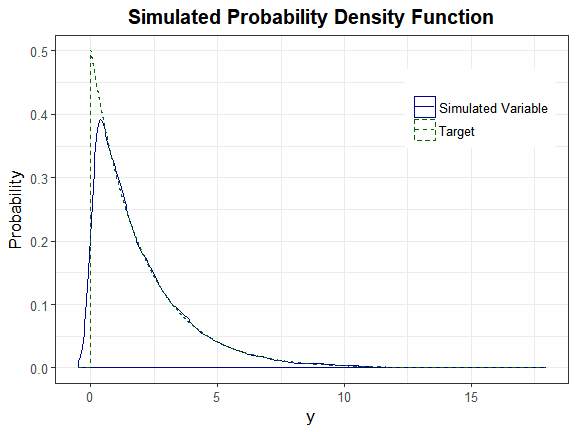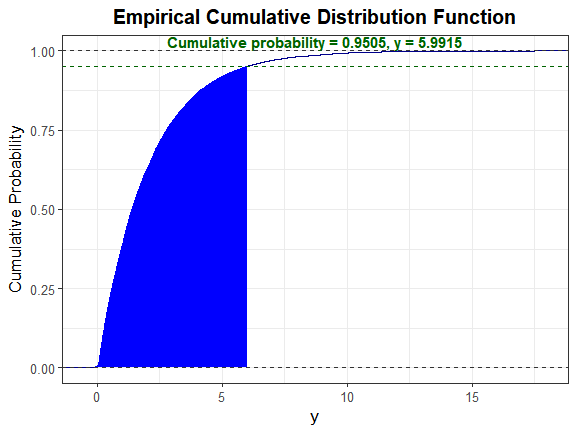Comparison of Simulated Distribution to Theoretical Distribution or Empirical Data

2018-06-28

Headrick and Kowalchuk (2007) outlined a general method for comparing a simulated distribution $$\Large Y$$ to a given theoretical distribution $$\Large Y^*$$. Note that these could easily be modified for comparison to an empirical vector of data:

1. Obtain the standardized cumulants (skewness, kurtosis, fifth, and sixth) for $$\Large Y^*$$. This can be done using calc_theory along with either the distribution name (plus up to 4 parameters) or the pdf fx (plus support bounds). In the case of an empirical vector of data, use calc_moments or calc_fisherk.

2. Obtain the constants for $$\Large Y$$. This can be done using find_constants or by simulating the distribution with nonnormvar1.

3. Determine whether these constants produce a valid power method pdf. The results of find_constants or nonnormvar1 indicate whether the constants yield an invalid or valid pdf. The constants may also be checked using pdf_check. If the constants generate an invalid pdf, the user should check if the kurtosis falls above the lower bound (using calc_lower_skurt). If yes, a vector of sixth cumulant correction values should be used in find_constants or nonnormvar1 to find the smallest correction that produces valid pdf constants.

4. Select a critical value from $$\Large Y^*$$, i.e. $$\Large y^*$$ such that $$\Large Pr(Y^* \ge y^*) = \alpha$$. This can be done using the appropriate quantile function and $$\Large 1 - \alpha$$ value (i.e. qexp(1 - 0.05)).

5. Solve $$\Large m_{2}^{1/2} * p(z') + m_{1} - y^* = 0$$ for $$\Large z'$$, where $$\Large m_{1}$$ and $$\Large m_{2}$$ are the 1st and 2nd moments of $$\Large Y^*$$.

6. Calculate $$\Large 1 - \Phi(z')$$, the corresponding probability for the approximation $$\Large Y$$ to $$\Large Y^*$$ (i.e. $$\Large 1 - \Phi(z') = 0.05$$) and compare to the target value $$\Large \alpha$$.

7. Plot a parametric graph of $$\Large Y^*$$ and $$\Large Y$$. This can be done with a set of constants using plot_pdf_theory (overlay = TRUE) or with a simulated vector of data using plot_sim_pdf_theory (overlay = TRUE). If comparing to an empirical vector of data, use plot_pdf_ext or plot_sim_pdf_ext.

Example

Use these steps to compare a simulated exponential(mean = 2) variable to the theoretical exponential(mean = 2) density. (Note that the printr package is invoked to display the tables.)

Step 1: Obtain the standardized cumulants

In R, the exponential parameter is rate <- 1/mean.

library("SimMultiCorrData")
library("printr")
stcums <- calc_theory(Dist = "Exponential", params = 0.5)

Step 2: Simulate the variable

Note that calc_theory returns the standard deviation, not the variance. The simulation functions require variance as the input.

H_exp <- nonnormvar1("Polynomial", means = stcums, vars = stcums^2,
skews = stcums, skurts = stcums,
fifths = stcums, sixths = stcums, n = 10000,
seed = 1234)
## Constants: Distribution  1
##
## Constants calculation time: 0 minutes
## Total Simulation time: 0 minutes

Look at the power method constants.

as.matrix(H_exp$constants, nrow = 1, ncol = 6, byrow = TRUE) c0 c1 c2 c3 c4 c5 -0.3077396 0.8005605 0.318764 0.0335001 -0.0036748 0.0001587 Look at a summary of the target distribution. as.matrix(round(H_exp$summary_targetcont[, c("Distribution", "mean", "sd",
"skew", "skurtosis", "fifth",
"sixth")], 5), nrow = 1, ncol = 7,
byrow = TRUE)
Distribution mean sd skew skurtosis fifth sixth
mean 1 2 2 2 6 24 120

Compare to a summary of the simulated distribution.

##  "TRUE"

Step 4: Select a critical value

Let $$\Large \alpha = 0.05$$.

y_star <- qexp(1 - 0.05, rate = 0.5) # note that rate = 1/mean
y_star
##  5.991465

Step 5: Solve for $$\Large z'$$

Since the exponential(2) distribution has a mean and standard deviation equal to 2, solve $$\Large 2 * p(z') + 2 - y_star = 0$$ for $$\Large z'$$. Here, $$\Large p(z') = c0 + c1 * z' + c2 * z'^2 + c3 * z'^3 + c4 * z'^4 + c5 * z'^5$$.

f_exp <- function(z, c, y) {
return(2 * (c + c * z + c * z^2 + c * z^3 + c * z^4 +
c * z^5) + 2 - y)
}

z_prime <- uniroot(f_exp, interval = c(-1e06, 1e06),
c = as.numeric(H_exp$constants), y = y_star)$root
z_prime
##  1.644926

Step 6: Calculate $$\Large \Phi(z')$$

1 - pnorm(z_prime)
##  0.04999249

This is approximately equal to the $$\Large \alpha$$ value of 0.05, indicating the method provides a good approximation to the actual distribution.

Step 7: Plot graphs

plot_sim_pdf_theory(sim_y = H_exp$continuous_variable[, 1], Dist = "Exponential", params = 0.5)We can also plot the empirical cdf and show the cumulative probability up to y_star. plot_sim_cdf(sim_y = H_exp$continuous_variable[, 1], calc_cprob = TRUE,
delta = y_star)Calculate descriptive statistics.

as.matrix(t(stats_pdf(c = H_exp\$constants[1, ], method = "Polynomial",
alpha = 0.025, mu = stcums, sigma = stcums)))
trimmed_mean median mode max_height
1.858381 1.384521 0.104872 1.094213